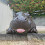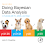## Friday, December 8, 2017

### Graphs of imputed censored y values

When using JAGS with censored data, the censored values are imputed to be consistent with the parameters of the model and the censoring limits. It's straight forward to record and graph the imputed values. Here are a couple of slides from my workshops that show how. Start by looking at Section 25.4 of DBDA2E, then the code below follows:

1.Thanks for the quick and complete answer. It worked like a charm!

Nice trick where you only graph the first imputed y from each interval since all the censored y's within each region will look the same.

- Sean

1.In general, each censored datum can have its own censoring cutoff, so the code I included in the post might not work generally. But at least it gives the idea... Glad it helped.

2.I'm now attempting to implement a missing predictor model using the HtWt30 robust regression example.

It seems like I'm close, but the model won't quite run. Right now I get a runtime error: unable to resolve the following parameters: x, x, x line 5, and so on. I created a prior for use when the x's are missing so I'm not sure what's causing the error.

Note that the missingIdx data is a column where mIdx[i]>0 for missing x values. Also note that I hard-coded some of the values for convenience that should be calculated on the fly.

I also apologize in advance for the terrible formatting of the code. I couldn't figure out how to get blogspot to let me paste in html code.

the code:
genMCMC = function( data, xName="x", yName="y", missingIdx="missingIdx", numSavedSteps=50000, saveName=NULL ) {
#-----------------------------------------------------------------------------
# THE DATA.
y = data[,yName]
x = data[,xName]
meanY = mean(y)
meanX = mean(x,na.rm=TRUE)
sdY = sd(y)
sdX = sd(x,na.rm=TRUE)
mIdx = data[,missingIdx] # > 0 when predictor missing
Ntotal = length(y)
# Specify the data in a list, for later shipment to JAGS:
dataList = list(
x = x ,
y = y ,
mIdx = mIdx ,
meanY = meanY ,
meanX = meanX ,
sdY = sdY ,
sdX = sdX ,
Ntotal = Ntotal
)
#-----------------------------------------------------------------------------
# THE MODEL.
modelString = "
# Standardize the data:
data {
for ( i in 1:Ntotal ) {
zx[i] <- ifelse ( mIdx[i]==0, (x[i]-meanX)/sdX , x[i] ) # skip NA's
zy[i] <- ( y[i] - meanY ) / sdY
}
}
# Specify the model for standardized data:
model {
for ( i in 1:Ntotal ) {
zy[i] ~ dt( zbeta0 + zbeta1 * zx[i] , 1/zsigma^2 , nu )
}
# prior used for missing zx's
zx ~ dnorm( 0 , 1 )
# Priors vague on standardized scale:
zbeta0 ~ dnorm( 0 , 1/(10)^2 )
zbeta1 ~ dnorm( 0 , 1/(10)^2 )
zsigma ~ dunif( 1.0E-3 , 1.0E+3 )
nu ~ dexp(1/30.0)
# Transform to original scale:
beta1 <- zbeta1 * ysd / xsd
beta0 <- zbeta0 * ysd + ym - zbeta1 * xm * ysd / xsd
sigma <- zsigma * ysd
x <- zx*sdX + meanX
}
" # close quote for modelString
writeLines( modelString , con="TEMPmodel.txt" )
#-----------------------------------------------------------------------------
# INITIALIZE THE CHAINS
# Initial values of MCMC chains based on data:
beta0 = 0 ; zbeta0 = 0
beta1 = 3.6440 # coef(lm(y~x))["x"]
zbeta1 = 0.5295 # zbeta1 = cor(zx, zy, use = "complete.obs")
sigma = sd(y) # na.rm=TRUE is default
zsigma = 1
nu = 30
# initial values for missing data:
xInit = rep( NA , length(x) )
for ( i in 1:length(y) ) {
if ( mIdx[i] > 0 ) { # only initialize if x is missing
xInit[i] = 56.357662 + 0.0690965*y[i] # hardcoded to keep it simple
}
}
initsList = list( beta0=beta0, beta1=beta1, zbeta0=zbeta0, zbeta1=zbeta1, zsigma=zsigma, sigma=sigma, nu = nu, x=xInit )
#-----------------------------------------------------------------------------
# RUN THE CHAINS
parameters = c( "beta0", "beta1", "sigma", "zbeta0", "zbeta1", "zsigma", "nu", "x")

# including "x" allows us to see imputed x distributions

1.JAGS can't impute missing predictor values because there is no model of the predictor values. In fact, at missing predictor values JAGS can't compute a mean for the predicted values either. If you have missing predictor values, you need a model that simultaneously models the predictor and predicted values (which basically makes both of them predicted values). And no, I don't have any examples --- sorry!

3.In the spirit of starting with a simple model, getting it to work, and then layering on the complexity, I was trying to get the simplest missing predictor case to work (one predictor, one outcome, 6/30 missing predictor values). I assumed the missing predictors were centered around x=0 with a std dev of 1 on the standardized scale. Initial values were determined by the inverse prediction equation x=f(y) from the complete data. Of course the imputed predictor values should be close to those initial values, but I'm really just trying to get the HtWt30 model to work with those missing predictors before I move onto the harder cases.

Conceptually isn't imputing a few missing predictor values as the model is MCMC'ed similar to jointly determining the regression model and the inverse prediction?

In my real case study, I do have intermediate measurements that will inform the missing predictor values. I have 10 predictors all with some "true" measurements and some missing measurements (missing values that will be independently measured - i.e. observed values measured with unknown error) with intermediate values to dial them in. That model seems like a bear to program/debug. I could have started with the multiple predictor case (like the Guber data) with one of the predictors missing a few values since that would be more realistic, but I truly wanted to start with the simplest of the simple missing value cases.

1.For univariate y and multiple x, there is no inverse equation x=f(y). You need a model of x. One approach is to describe the joint distribution of y,x with a multivariate normal... but that might not be a good description of the data.

4.Do you have any examples of a model with some missing predictor values?

I would assume there are cases like a repeated measurement experiment in medicine where imputing a missing data point is more helpful than throwing out all the incomplete cases. The posterior of those missing data points may be wide, but you try to extract maximum information from the datapoints you do have (given the assumptions of missing at random, multivariate normal, etc).

1.Well, there's the multivariate normal that I mentioned previously. The topic of modeling missing data is enormous, and deserves a book of its own. There are layers of complications, the first being whether the data are missing completely at random, or whether the missingness is itself a function of the values of the other variables. If missingness is not at random, the systematicity needs to be modeled. Then there's choosing the model itself. Do you want each missing value to be a function of all the other variables? Of a subset of the other variables? What sort of noise distribution? All of these choices could be informed by domain-specific theoretical considerations.